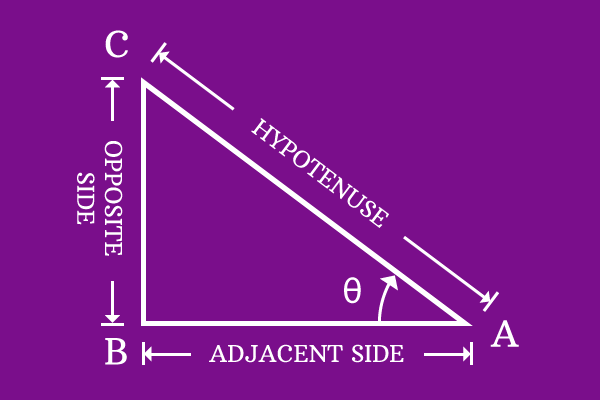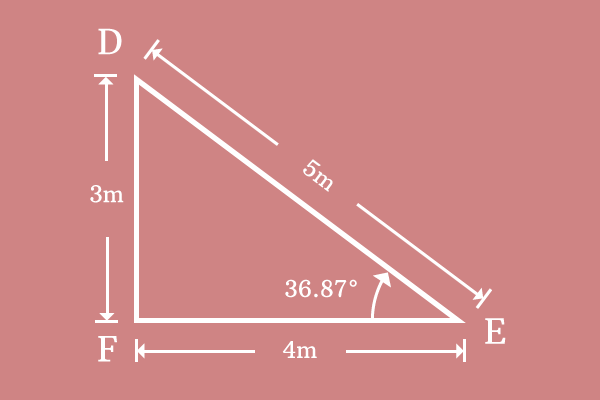# Cosine## Definition

The ratio of lengths of adjacent side to hypotenuse at the associated angle of the right angled triangle is called cosine.

Cosine apprises the direct relation of angle with ratio of ratio of length of adjacent side to length of hypotenuse in the right angled triangle. Due to this reason, cosine is also called as a trigonometric function in trigonometry.

### Expression

Cosine is written as a ratio of length of adjacent side to length of hypotenuse in mathematical form.

$$\frac{Length \, of \, Adjacent \, side}{Length \, of \, Hypotenuse}$$The ratio is calculated at a particular angle of the right angled triangle. Therefore, the relation of angle with ratio in a right angled triangle is written as cosine of angle. Cosine of angle is expressed in mathematics by writing $\cos$ first and then angle of the triangle.

The relation of angle with ratio of lengths of adjacent side to hypotenuse is written in mathematical form as follows.

$$\cos \, (angle) = \frac{Length \, of \, Adjacent \, side}{Length \, of \, Hypotenuse}$$

Cosine is defined mathematically in ratio form in trigonometry. So, it is known as a trigonometric ratio.

For example, $\Delta CAB$ is a right angled triangle and its angle is $\theta$. Lengths of adjacent side and hypotenuse are $AB$ and $AC$ respectively.

$$\therefore \,\, cos \, \theta = { AB \over AC }$$

#### ExampleIn $Δ DEF$, the lengths of adjacent side, hypotenuse and angle are measured and they are.

1. The length of adjacent side $(\overline{EF})$ is $EF = 4$ meters
2. The length of hypotenuse $(\overline{DE})$ is $DE = 5$ meters
3. The angle of right angled triangle $DEF$ is $36.87^°$.

$$cos \, 36.87^° = { EF \over DE }$$

Substitute these three values in the expression of cosine.

$$cos \, 36.87^° = { 4 \over 5 }$$

$⇒ \, cos \, 36.87^° = 0.8$

The value of ratio of length of adjacent side to length of hypotenuse is $0.8$ at angle $36.87^°$. It is called as the value of cosine at angle $36.87^°$. In this way, cosine represents the relation of angle with ratio of length of adjacent side to length of hypotenuse in a right angled triangle.

Latest Math Topics
Latest Math Problems
Email subscription
Math Doubts is a best place to learn mathematics and from basics to advanced scientific level for students, teachers and researchers. Know more